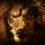# Probability Question

Hi people! I'm new to probability and so while going through conditional probability I found the following problem which I solved by arguing directly without using any results of Conditional Probability ............unfortunately the answer wasn't given. The problem goes as:

There are 4 friends A,B,C, D and a referee. A puts a '+' or a '-' sign on a piece of paper and passes it to B who perhaps changes the sign and passes the slip to C, who may change the sign and passes it to D. D perhaps changes the sign and finally passes the slip to the referee. Suppose 2/3 is the prob. of writing a '+' by A and each of B,C,D changes the sign by prob 1/3 independently. If the referee notices a '+' sign on the slip, then find the prob that A wrote a '+' on the slip.

I would really appreciate if someone gave me the solution. Also assuming the same conditions, what would then be the ans, instead of 4 friends if n friends did the same thing.Note by Kulkul Chatterjee
6 years, 7 months ago

This discussion board is a place to discuss our Daily Challenges and the math and science related to those challenges. Explanations are more than just a solution — they should explain the steps and thinking strategies that you used to obtain the solution. Comments should further the discussion of math and science.

When posting on Brilliant:

• Use the emojis to react to an explanation, whether you're congratulating a job well done , or just really confused .
• Ask specific questions about the challenge or the steps in somebody's explanation. Well-posed questions can add a lot to the discussion, but posting "I don't understand!" doesn't help anyone.
• Try to contribute something new to the discussion, whether it is an extension, generalization or other idea related to the challenge.

MarkdownAppears as
*italics* or _italics_ italics
**bold** or __bold__ bold
- bulleted- list
• bulleted
• list
1. numbered2. list
1. numbered
2. list
Note: you must add a full line of space before and after lists for them to show up correctly
paragraph 1paragraph 2

paragraph 1

paragraph 2

[example link](https://brilliant.org)example link
> This is a quote
This is a quote
    # I indented these lines
# 4 spaces, and now they show
# up as a code block.

print "hello world"
# I indented these lines
# 4 spaces, and now they show
# up as a code block.

print "hello world"
MathAppears as
Remember to wrap math in $$ ... $$ or $ ... $ to ensure proper formatting.
2 \times 3 $2 \times 3$
2^{34} $2^{34}$
a_{i-1} $a_{i-1}$
\frac{2}{3} $\frac{2}{3}$
\sqrt{2} $\sqrt{2}$
\sum_{i=1}^3 $\sum_{i=1}^3$
\sin \theta $\sin \theta$
\boxed{123} $\boxed{123}$

Sort by:

This is my first impression of how to solve it. I'm not sure my numbers are all right, but I'm fairly sure this should be the approach:

The chance that a referee saw '+' is the chance that it was '+' originally and was changed 0 or 2 times, or the chance that it was '-' originally and was changed 1 or 3 times.

The first of these has chance $2/3$ (chance that A wrote '+') times the sum of $(2/3)^3$ and $3\cdot(1/3)^2\cdot 2/3$ (chance that it was changed 0 times or 2 times). Thus, the chance that A wrote '+' and the referee saw '+' is equal to $28/81$.

The second has chance $1/3$ (chance that A wrote '-') times the sum of $(1/3)^3$ and $3\cdot(2/3)^2 \cdot 1/3$ (chance that it was changed 3 times or 1 time). This chance is $12 / 81$.

Thus, the total chance that the referee saw '+' is $40/81$. The chance that the referee saw '+' and A wrote '+' is $28/81$, so the chance that A wrote '+' given the referee saw '+' is $(28 / 81) / (40 / 81) = 7 / 10$.(Note the general identity $P(A \ \mathrm{and}\ B) = P(A) \cdot P(B | A)$, in this case $A$ is that the referee saw '+' and $B$ is the chance that A wrote '+.')

To generalize this, note that the chance that the number of changes is odd is 1 minus the chance that the number of changes is even. The only difficulty is calculating one without knowing the other. Since the chance of changing is not $1/2$, the sum cannot be collapsed due to symmetry. If there are $n$ middlemen (in this case, $n = 3$), then the chance of no changes is given by the first, third, etc. terms in the binomial expansion of $(2/3 + 1/3)^n$. The eventual answer will be the quotient of $2/3$ times the even change chance and the sum of $2/3$ times the even change chance plus $1/3$ times the odd change chance.

- 6 years, 7 months ago

Thanks Alexander, thanks a lot!........My solution was HIGHLY incorrect!!

- 6 years, 7 months ago

Good question man............

- 6 years, 7 months ago